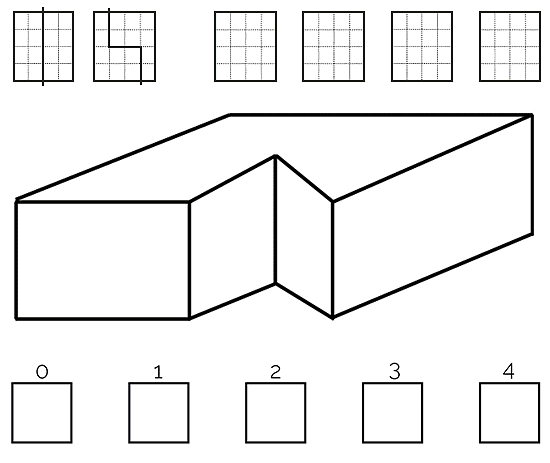## A Few Challenges:A  Straight forward:
A1 A square of paper is marked out into sixteen smaller squares. There are six ways of cutting the square along these marks into two pieces of the same size and shape?

Two ways are shown right, can you find the other four ways?

A2 If four mice can eat four pounds of cheese in four minutes; How long will it take 99 mice to eat 99 pounds of cheese?

A3 Thirty eight children are seated, equally spaced, around a large circular table. They are numbered in order from 1 up to 38. What is the number of the child opposite child number 8?

B More Interesting:
B1 How many times each day do the hands of a clock point in exactly opposite directions?

B2 Two men were travelling with a dog. A’s walking speed is 3 km per hour. B’s walking speed is 6 km per hour. One morning A left the hotel by himself and walked down a road for 3 km before B left the hotel with the dog. The dog playfully trotted back and forth between the two men at a speed of 10 km per hour. How many kilometres had the dog run by the time B caught up with A?

B3 A square cake, shown right, is to be cut into slices in the way that round cakes are usually cut – i.e. each cut is from the centre straight out to the edge:

It would be easy to divide the cake into 4 equal parts. But how would you cut the whole cake into 3 equal parts? (With 3 straight cuts from the centre to the edge.)

C Challenging:
C1 These five boxes, shown right, are labelled from 0 to 4:

Your challenge is to put a single digit number into each box so that:
• the number in box zero is the number of boxes containing zero.
• the number in box one is the number of boxes containing a one.
• the number in box two is the number of boxes containing a two etc.

C2 Twelve coins look identical but one of them is a fake. The fake coin is a different weight but looks the same as the other eleven. You have a simple beam balance which will balance if two piles of coins weigh the same or it will show which pile is heavier or lighter. How can you use just three weighings to identify the fake coin and discover whether it is lighter or heavier than a proper coin?

C3 Is there a way to divide up any obtuse angled triangle into smaller triangles which are all acute angled triangles?

(Obtuse angled have one angle greater than 90° while acute angled triangles have all their angles less than 90°. No right angles allowed!)

Visit www.yoursteyning.co.uk web site for the answers.